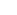How Cheenta works to ensure student success?
Explore the Back-Story

# Are juniors countable if seniors are?: TIFR GS 2018 Part A Problem 21# Understand the problem

A countable group can have only countably many distinct subgroups.

Hint 1
This is a really artistic problem.
Pre-Solution Thoughts:
Lemma: A group is finite iff the number of subgroups of a group is finite.(Check!)
The lemma is a way to understand that an infinite group will have infinite number of subgroups.

Hint 2
• We are considering here set of all subsets of a countable group G, which is a subset of the Power Set of G.
• Given G is countable the Power Set of G is uncountable.
• Now we know that, where d is the set of natural numbers denotes the cardinality of the Power Set of G which is an uncountable set as it is bijective to the Real Numbers [Here d is not finite by the way, d is countably infinite].
• So it is kinda intuitive that it may be uncountable.
• First I took the group (Z,+), but we all know that the subgroups of  Z are nZ only. This doesn’t solve our purpose.
• So naturally the next choice was the group (Q.+) whose subgroup is (Z,+).
• While understanding the subgroups of (Q.+), the question is solved.
• Hint 3
• We need to understand the subgroups of (Q.+).
• Consider any rational number q and the subgroup qZ of (Q.+) generated by q.
• What if we take two rational numbers?
• For simplicity check the subgroup generated by {1/2 , 1/3}.
• Prove that the subgroup generated by {1/2 , 1/3} is (1/2.3)Z.{Observe that co-prime property of 2 and 3 is playing an important role}.
• Hint 4
• Now what if we take three mutually coprime natural numbers say a,b,c and see the subgroup generated by { 1/a , 1/b ,1/c}. In fact for simplicity take a,b,c to be primes.
• Observe that it is of the form (1/a.b.c)Z.
• Hence for every finite subset of primes, we generate a distinct subgroup of (Q,+).
• Hence the total number of subgroups contained all the subsets of primes, which has a bijection with the Real Numbers as mentioned above.
• So the answer is False.
• Exercise
• Find all the subgroups of (Q,+) with proof.

# Connected Program at Cheenta

The higher mathematics program caters to advanced college and university students. It is useful for I.S.I. M.Math Entrance, GRE Math Subject Test, TIFR Ph.D. Entrance, I.I.T. JAM. The program is problem driven. We work with candidates who have a deep love for mathematics. This program is also useful for adults continuing who wish to rediscover the world of mathematics.

# Understand the problem

A countable group can have only countably many distinct subgroups.

Hint 1
This is a really artistic problem.
Pre-Solution Thoughts:
Lemma: A group is finite iff the number of subgroups of a group is finite.(Check!)
The lemma is a way to understand that an infinite group will have infinite number of subgroups.

Hint 2
• We are considering here set of all subsets of a countable group G, which is a subset of the Power Set of G.
• Given G is countable the Power Set of G is uncountable.
• Now we know that, where d is the set of natural numbers denotes the cardinality of the Power Set of G which is an uncountable set as it is bijective to the Real Numbers [Here d is not finite by the way, d is countably infinite].
• So it is kinda intuitive that it may be uncountable.
• First I took the group (Z,+), but we all know that the subgroups of  Z are nZ only. This doesn’t solve our purpose.
• So naturally the next choice was the group (Q.+) whose subgroup is (Z,+).
• While understanding the subgroups of (Q.+), the question is solved.
• Hint 3
• We need to understand the subgroups of (Q.+).
• Consider any rational number q and the subgroup qZ of (Q.+) generated by q.
• What if we take two rational numbers?
• For simplicity check the subgroup generated by {1/2 , 1/3}.
• Prove that the subgroup generated by {1/2 , 1/3} is (1/2.3)Z.{Observe that co-prime property of 2 and 3 is playing an important role}.
• Hint 4
• Now what if we take three mutually coprime natural numbers say a,b,c and see the subgroup generated by { 1/a , 1/b ,1/c}. In fact for simplicity take a,b,c to be primes.
• Observe that it is of the form (1/a.b.c)Z.
• Hence for every finite subset of primes, we generate a distinct subgroup of (Q,+).
• Hence the total number of subgroups contained all the subsets of primes, which has a bijection with the Real Numbers as mentioned above.
• So the answer is False.
• Exercise
• Find all the subgroups of (Q,+) with proof.

# Connected Program at Cheenta

The higher mathematics program caters to advanced college and university students. It is useful for I.S.I. M.Math Entrance, GRE Math Subject Test, TIFR Ph.D. Entrance, I.I.T. JAM. The program is problem driven. We work with candidates who have a deep love for mathematics. This program is also useful for adults continuing who wish to rediscover the world of mathematics.

# Similar Problems

This site uses Akismet to reduce spam. Learn how your comment data is processed.

### Knowledge Partner